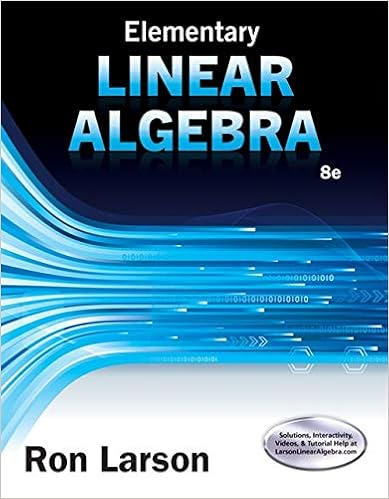# Y y a x a x y a x r r x y y r experiment 2 2suspend a

• Notes
• 4
• 50% (6) 3 out of 6 people found this document helpful

This preview shows page 2 - 4 out of 4 pages.

##### We have textbook solutions for you!
The document you are viewing contains questions related to this textbook.The document you are viewing contains questions related to this textbook.
Chapter 4 / Exercise 60
Elementary Linear Algebra
LarsonExpert Verified
YyAxAxyAxRRxyyR
##### We have textbook solutions for you!
The document you are viewing contains questions related to this textbook.The document you are viewing contains questions related to this textbook.
Chapter 4 / Exercise 60
Elementary Linear Algebra
LarsonExpert Verified
Experiment 22)Suspend a mass of 100 g at 300 and a mass of 200 g at 1200. Find the resultant of these two forces by the method of components. Check your result by mounting a pulley directly opposite to the resultant and of same magnitude; the ring should be centered.3)Keep the same masses above listed and suspend a third mass of 150 g at 2300.Do the same thing as in procedure 2.- 3 -
Experiment 2Report formName______________Date __________Calculations:1)For procedure 1, show your calculation for the components of the vector.2)For procedure 2, find, graphically, the magnitude and the direction of the resultant vector.Use the laws of sine and cosine. Show your graph to scale on a separate sheet, if needed. .3)For procedure 2, find, analytically, the magnitude and the direction of the resultant vector.Use the method of components.4)For procedure 3, find, analytically, the magnitude and the direction of the resultant vector.Use the method of components.Post Lab Questions:1) State in your own words what your conclusion is from this experiment.2)What are the main roles of the pulleys? Explain.3) Can the ring be in equilibrium while all the forces acting on it lie in two adjacent plane? Explain.4) State the condition for translational equilibrium of a particle.5) What are some possible sources of error? - 4 -
•••